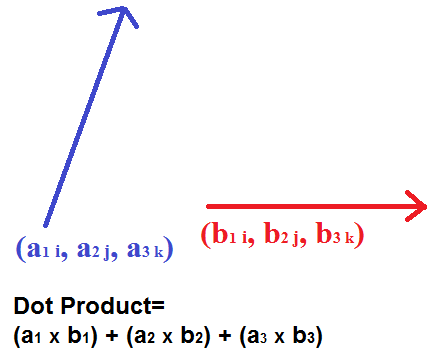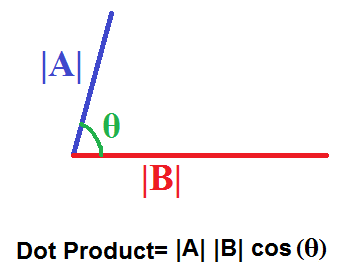﻿ Dot Product Calculator ﻿# Dot Product Calculatora1: i a2: j a3: k b1: i b2: j b3: k

0

This Dot Product calculator calculates the dot product of two vectors based on the vector's position and length.

This calculator can be used for 2D vectors or 3D vectors. If a user is using this vector calculator for 2D vectors, which are vectors with only two dimensions, then s/he only fills in the i and j fields and leave the third field, k blank. If using this calculator for a 3D vector, then the user enters in all fields.

The dot product of the two vectors which are entered are calculated according to the formula shown above. The i, j, and k fields are multiplied together and then all values are added up to give the total dot product.

The resultant of this calculation is a scalar. The dot product merely finds the total length of the two vectors as just length, not direction. Thus, the result is a scalar, not a vector.

### Dot Product Calculator By Magnitude and Cosine Angle|A|: |B|: θ:

0

This Dot Product calculator calculates the dot product of 2 vectors by the value of the magnitudes of the 2 vectors and the angle between them.

Unlike the first calculator, which calculated the dot product by each of the vector's dimensions on the i, j, and k planes, here the dot product is calculated by the total magnitudes of the vectors multiplied by the cosine of the angle between them, as shown in the formula above.

Just like the first calculator, this one can be utilized for 2D or 3D vectors. If 2D or 3D, just place the magnitudes of each of the vectors, and the respective dot product calculation will be computed.

Again, the dot product calculates a scalar result, the length of the vectors added together. The resultant is not a vector, but simply the magnitude of the addition of the 2 vectors.

### Dot Product Explained

First to explain what it is, the dot product is the summation of 2 vectors, such that the resultant value is the length of the 2 vectors as if both vectors were added up.

There are 2 ways (2 formulas) to compute the dot product of 2 vectors.

Way 1

If you have the values of all the dimensions of the 2 vectors, meaning of all the planes (x, y, z), then you can compute the dot product by the following method or formula:

Dot Product= (a1 * b1) + (a2 * b2) + (a3 + b3)

Using this formula we simply multiply like terms (of the various planes) and add them all up to get our resultant value. Since we compute simply the length of the vectors added up, the result is simply a number, a scalar value. The result is not a vector.

Way 2

The second way to calculate the dot product is if you have the magnitudes of the 2 vectors and the value of the angle which is between them. Using this method, we compute the value by the following formula:

Dot Product= |A||B|cos(θ)

Using this formula, we just multiply the absolute values of the magnitudes of the 2 vectors with the cosine of the angle between them. We use absolute values for the magnitudes because the lengths cannot be negative. There can only be positive length. The dot product, however, may be negative if the vectors point away from each other, which is the case when the θ of cosine is between 90° and 270°. When vectors point opposite of each other, they don't add; they subtract. When cosine is between 0° and 90° and 270° and 360°, the vectors point in the same direction, so they add up.

Related Resources

﻿